## Technical Analysis Crosses in Data Mining

This tutorial assumes that you have an understanding of the basics of Data Mining in ShareScope. If you are not familiar with Data Mining then we recommend that you start by watching the Basic Data Mining video here.

In this tutorial:

#### Technical Analysis crosses: an introduction

A technical analysis cross occurs when an indicator crosses the price line, another indicator or its own signal line (typically a moving average of the indicator).

Regardless of the type of cross you are looking for, there are two basic concepts to consider:

##### Concept 1: Relative positions

The image below shows the blue line crossing above the red line: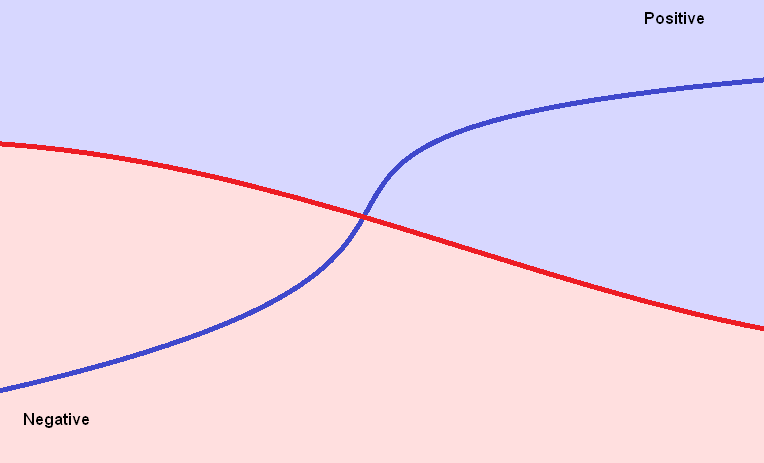Put another way, the blue line has moved from a negative position relative to the red line, to a positive position relative to the red line.

Expressed in simple mathematical terms:

If the blue line is below the red line on a chart then it has a smaller value.

i.e.

Smaller value (blue line) - Larger value (red line) = Negative result

If the blue line is above the red line (as it is after the lines have crossed), then it has a larger value.

i.e.

Larger value (blue line) - Smaller value (red line) = Positive result

When filtering for crosses in Data Mining, it is recommended to use trading days, rather than calendar days, to ensure that weekends and public holidays are excluded.

Depending on when you are using ShareScope, the most recent price data will either be the previous trading day or today (if viewing after the 6pm update on a trading day).

In Data Mining, 0 trading days ago to represent the most recent trading day in the database and 1 trading day ago represents the previous trading.

#### Example filter: Golden Cross

The Golden Cross occurs where a shorter-period moving average crosses above a longer-period moving average. In this example, we will filter for the 25 day MA crossing above the 100 day MA.

To create this, first open the Data Mining window (the toolbar button is denoted by the pick-axe and shovel; alternatively, select Change window/New window : Data Mining from the View menu).

Click on the Select Filter button and then select New filter. At this point you will be asked to name the new filter. We have called the filter 25/100SMA GLD Cross.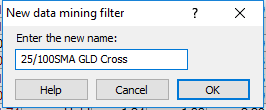Note: filter names can only be a maximum of 24 characters in length including spaces so some abbreviation in the filter name may be necessary.

This filter requires two criteria: one that looks at the pattern before the cross and one that looks at the pattern after the cross.

1. Click on the Add Criterion button and select the following options in the Data Mining Wizard:
2. Select Volume and Technical Analysis and click Next.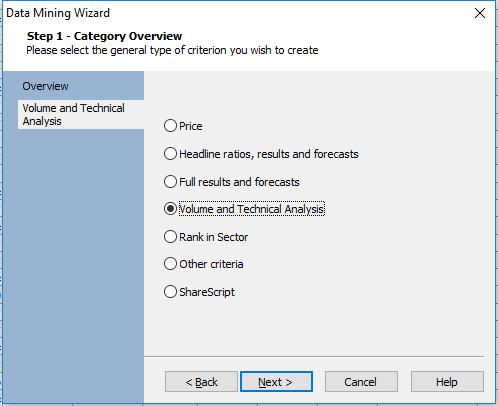3. Select Moving Average and click Next.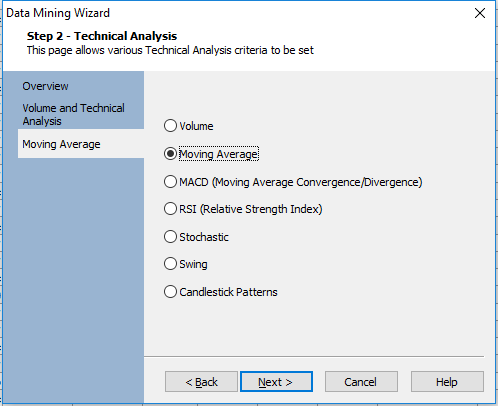4. Select Difference between two moving averages on date... and click Next.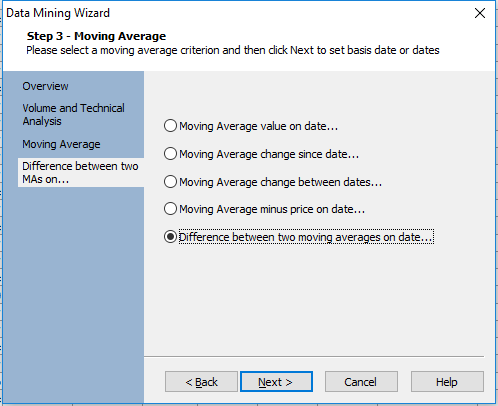5. Next, select Trading days, delete the default value of 20 from the box and replace it with 1. Then click Next.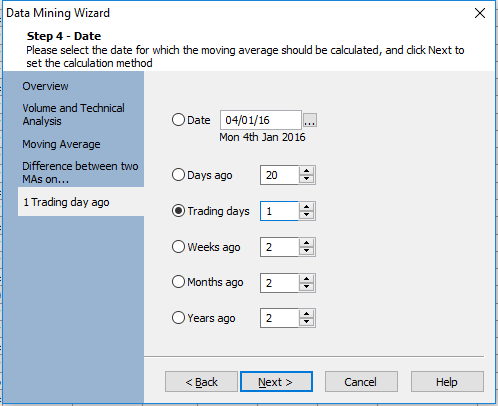6. In this example, we will use a simple moving average. All that remains is to enter the two moving average value: 25 and 100. Click Next to continue.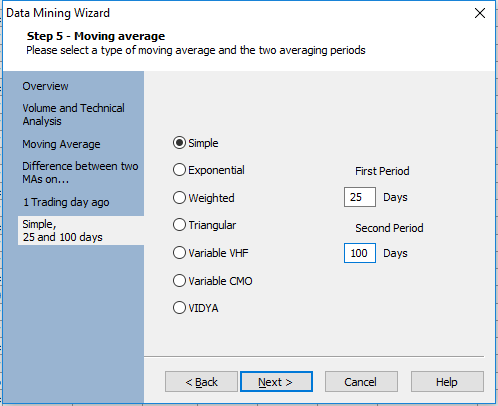7. Finally, select Percentage and click Finish.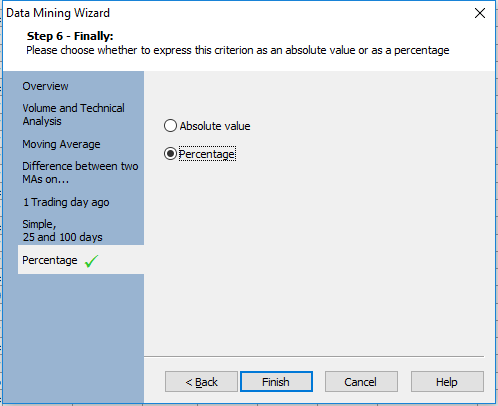8. The criterion is then added to the filter and will appear as follows: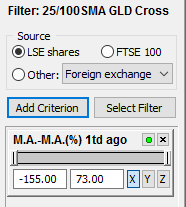The criterion has two values - a lower and an upper value. By default, this will encompass the full range of results for all of the shares in the current list.

This criterion is looking at the relationship between the two moving averages 1 trading day ago. As we want to find instances where the shorter-term moving average (25) starts lower than the longer-term moving average (100), the range should be negative.

To reflect this on the criterion we delete the right-hand value, which is positive, and replace it with -0.01.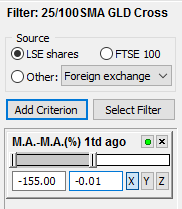The next thing to do is add a second criterion for 0 trading days ago; much in the same way as before:

1. Press the Add Criterion button
2. Select Volume and Technical Analysis and click Next.
3. Select Moving Average and click Next.
4. Select Difference between two moving averages on date... and click Next.
5. Select Trading days = 0. Click Next.
6. Enter the two moving average values - 25 and 100 - and click Next.
7. Select Percentage and click Finish.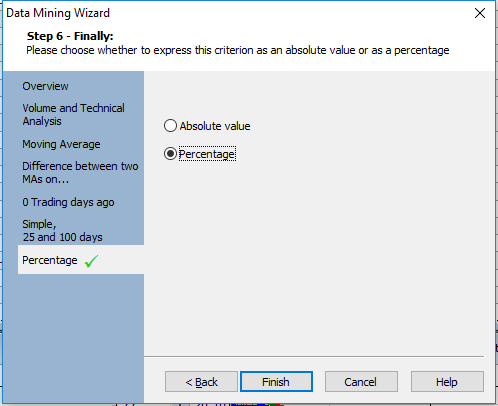A second criterion is added to the filter and, again, has values in the left and right-hand boxes representing the full range of results for the shares in the list.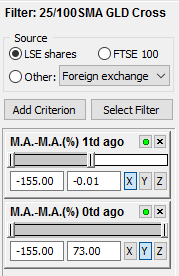This time we want to find where the shorter-term moving average is higher than the longer-term moving average - in other words, it has just crossed above the line. Therefore, a positive range is required. To do this, simply replace the negative value in the left-hand box with 0.01. Press the keyboard Enter key to ensure that has been registered by the filter.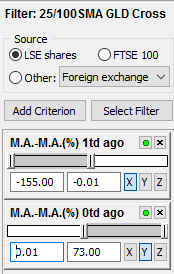This filter will scan for Golden Crosses occurring in the last trading day as illustrated below.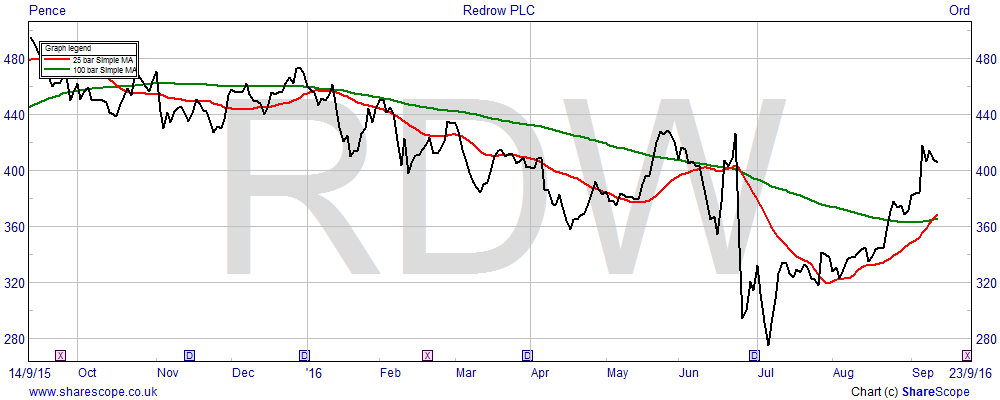It is also possible to look over a longer-period by changing the 1 trading day value. So, for example, we may want to find Golden Crosses occurring any time in the past 5 days. To do so, simply change the 1 trading day ago value to 4 (so we are looking at 0-4 trading days; 5 days in total).

A word of caution, however: whilst this will find all Golden Crosses over the past 5 days, it may also include shares where the moving averages have crossed more than once in this time period - for example, where the 25-day MA has crossed above the 100-day MA, before crossing back below it.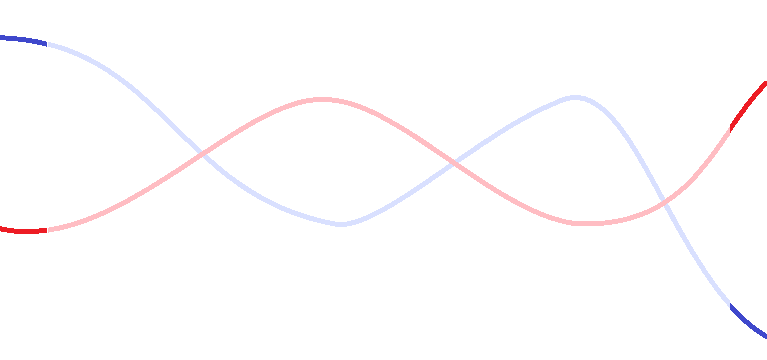Naturally, the greater the lookback period of the filter, the greater the likelihood of this occurrence - so it's important to inspect the chart for each of the shares returned by the filter.

A Dead Cross is the opposite of the Golden Cross: it looks for a shorter-term MA crossing below a longer-term one. The criteria used will be exactly the same, but the positive/negative filter values will need to be reversed.

In the case of a Dead Cross, the shorter-period MA starts above the longer-period MA (i.e. in a positive position) before moving below it (a negative position).

You can create a new filter as described in the previous section, or for ease, copy the entire Golden Cross filter before making the necessary changes to the filter values.

To copy the Golden Cross filter, right-click in the grey area below the two criteria boxes and select Copy filter. When prompted name the filter and press OK. Press Select filter to locate the new filter.

The criteria used are the same as for the Golden Cross, however, the first criterion (labelled M.A.-M.A.(%) 1td ago) needs to include a positive range, so replace the left-hand value with 0.01.

Then for the second criterion (labelled M.A.-M.A.(%) 1td ago), replace the right-hand value with -0.01.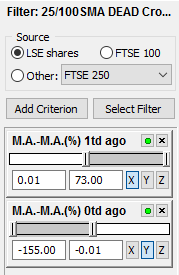#### Example filter: MACD crossover

To create a filter which scans for shares where the MACD has crossed above its signal line:

1. Click the New Filter button
3. Select Volume and Technical Analysis
4. Select MACD (Moving Average Convergence/Divergence)
5. Select MACD minus signal on date...
6. Select Trading days ago: 1
7. Amend the MACD and signal period if necessary.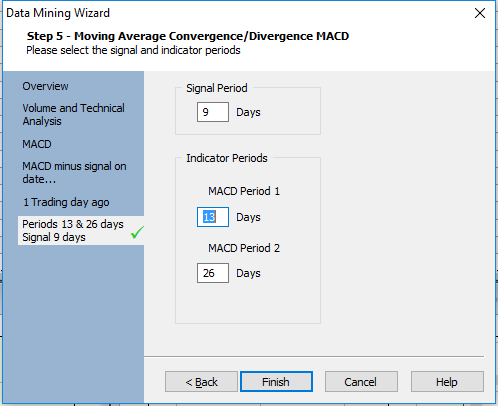Once added, change the criterion values as follows: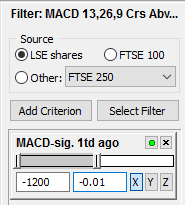Now for the second criterion:

2. Select Volume and Technical Analysis
3. Select MACD (Moving Average Convergence/Divergence)
4. Select MACD minus signal on date...
5. Select Trading days ago: 0
6. Amend the MACD and signal period figures if necessary. Make sure that these are the same as with the first criterion.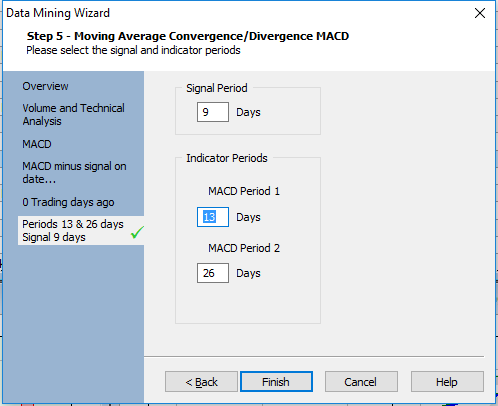Change the values of the second criterion as follows: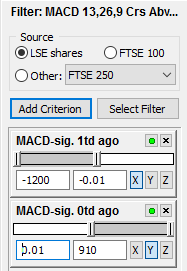To create a filter based on the MACD crossing below its signal line, we simply reverse the values, as shown below: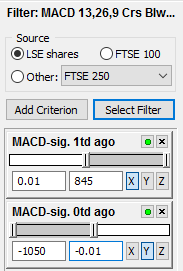#### Example filter: Stochastic Oscillator crossover

A Stochastic Oscillator crossover looks for the main indicator (%K) crossing above or below its signal line (%D).

Let's start with the Stochastic moving above its signal line. Create a new filter, select Add Criterion and then select the following options from the Data Mining Wizard:

1. Select Volume and Technical Analysis
2. Select Stochastic Oscillator
3. Select Indicator minus signal on date...
4. Select Trading days ago: 1
5. Change the Stochastic parameters if desired
6. Select Percentage and then click Finish.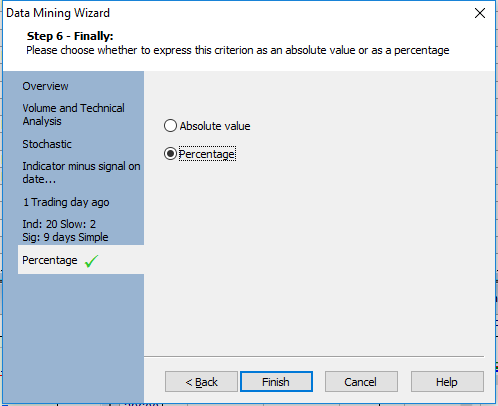Once added, change the values of the filter as follows: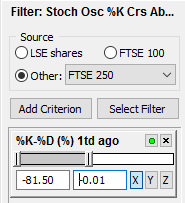Now for the second criterion:

1. Select Volume and Technical Analysis
2. Select Stochastic Oscillator
3. Select Indicator minus signal on date...
4. Select Trading days ago: 0
5. Change the Stochastic parameters if desired (make sure they match those in the first criteria)
6. Select Percentage and then click Finish.

Change the parameters of the second criterion as follows: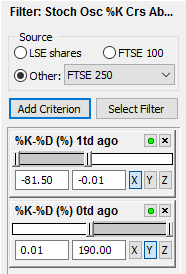To create the opposite filter - i.e. where the Stochastic falls below the signal line - simply reverse the figures: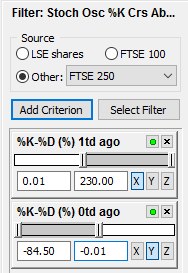#### Example filter: Price crossing a moving average

The final cross that we will cover is when the price crosses above, or below, a moving average.

To find shares where the price moves above a 25-day MA, create a new filter, select Add Criterion and then select the following from the Data Mining Wizard:

1. Select Volume and Technical Analysis
2. Select Moving Average
3. Select Moving average minus price on date...
4. Select Trading days ago: 1
5. Change the moving average value to 25
6. Select Percentage and press Finish.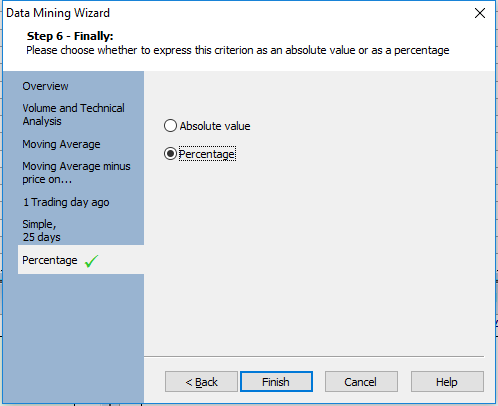The reason this filter was left until last is that the calculation involved is the opposite of the other crosses in this tutorial.

In this case, as per the title, the criterion is doing the sum: Moving average - Price

If the price is below the MA, it is the smaller value:

i.e.

Larger value (moving average) - Smaller value (price) = Positive result

And when the price moves above the MA, it is a larger value:

i.e.

Smaller value (moving average) - Larger value (price) = Negative result

This means that the 1 td ago criterion requires positive values: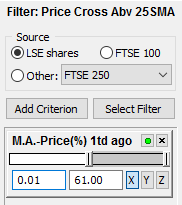Now for the second criteria:

1. Select Volume and Technical Analysis
2. Select Moving Average
3. Select Moving average minus price on date...
4. Select Trading days ago: 0
5. Change the moving average value to 25
6. Select Percentage and press Finish.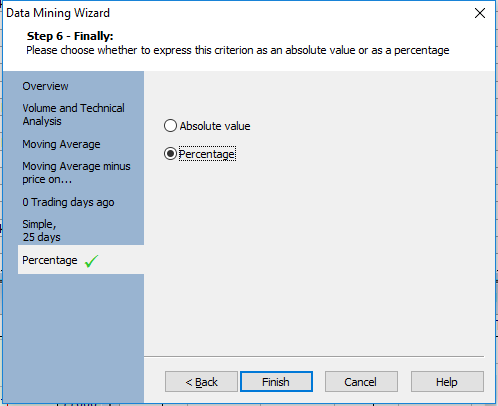The 0 td ago criterion requires a negative range, so: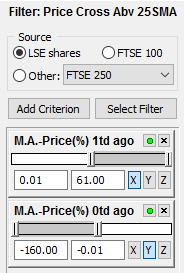The chart below show illustrate the black price line moving above the red 25-day MA.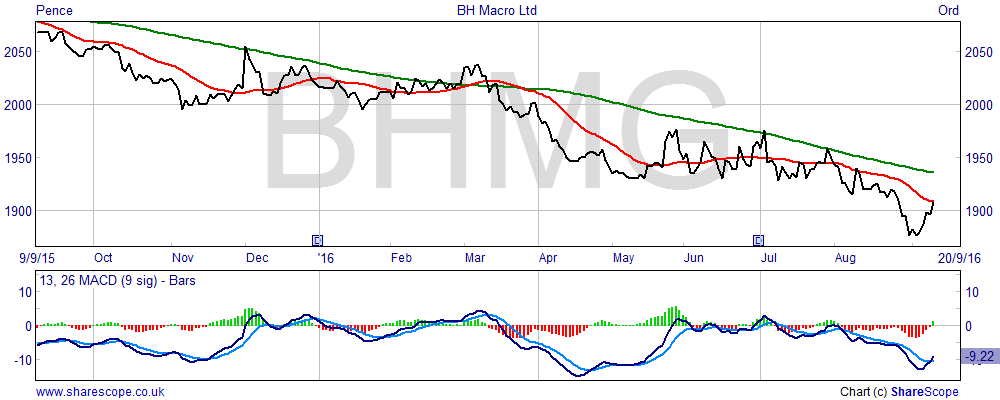To create a filter with the price falling below the moving average, the reverse logic is applied: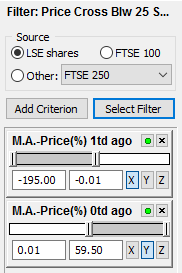Remember, if you have any trouble finding or using any of these features, please don't hesitate to contact our Customer Support team. They will be delighted to help.